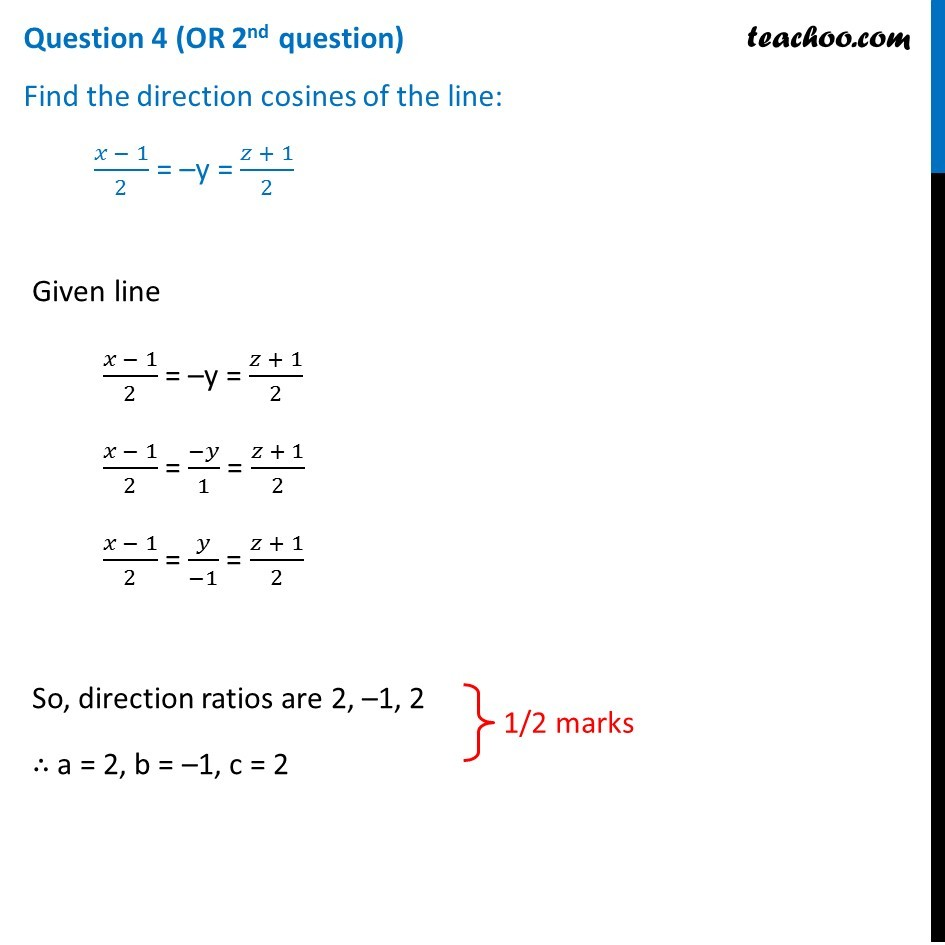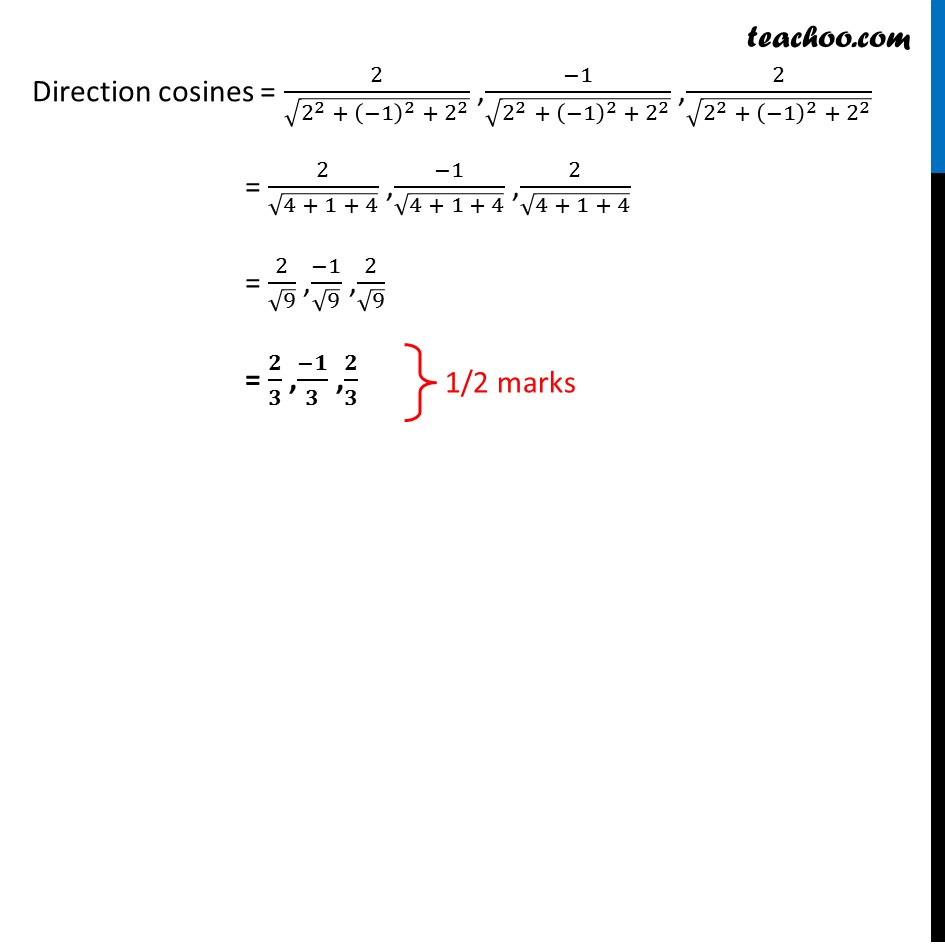Question 4 (OR 2 nd question)

Find the direction cosines of the line:

(x - 1)/2 = –y = (z + 1)/2Subscribe to our Youtube Channel - https://you.tube/teachoo

1. Class 12
2. Solutions of Sample Papers and Past Year Papers - for Class 12 Boards
3. CBSE Class 12 Sample Paper for 2019 Boards

Transcript

Question 4 (OR 2nd question) Find the direction cosines of the line: (𝑥 − 1)/2 = –y = (𝑧 + 1)/2 Given line (𝑥 − 1)/2 = –y = (𝑧 + 1)/2 (𝑥 − 1)/2 = (−𝑦)/1 = (𝑧 + 1)/2 (𝑥 − 1)/2 = 𝑦/(−1) = (𝑧 + 1)/2 So, direction ratios are 2, –1, 2 ∴ a = 2, b = –1, c = 2 Direction cosines = 2/√(2^2 + (−1)^2 + 2^2 ) ,(−1)/√(2^2 + (−1)^2 + 2^2 ) ,2/√(2^2 + (−1)^2 + 2^2 ) = 2/√(4 + 1 + 4) ,(−1)/√(4 + 1 + 4) ,2/√(4 + 1 + 4) = 2/√9 ,(−1)/√9 ,2/√9 = 𝟐/𝟑 ,(−𝟏)/𝟑 ,𝟐/𝟑

CBSE Class 12 Sample Paper for 2019 Boards

Class 12
Solutions of Sample Papers and Past Year Papers - for Class 12 Boards